# Alice Talks About Metric Measurement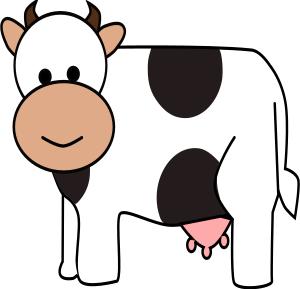Hi, everyone! If you haven't met me, my name is Alice (the cow), and I'm trying to help my nephew, Fred to learn all about how to measure things. Today, I thought I'd give him an introduction to metric units. I wonder if you can help me out.

Right, Fred. Humans like to count in powers of ten because they have ten fingers. The main powers of ten you need to know are 10, 100 and 1000. It's a bit trickier for us, with our hooves, but you'll eventually get used to it. I did!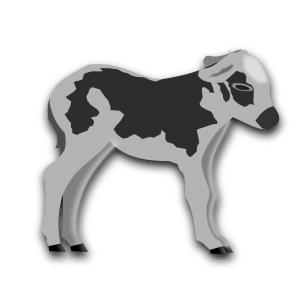## Measuring Liquids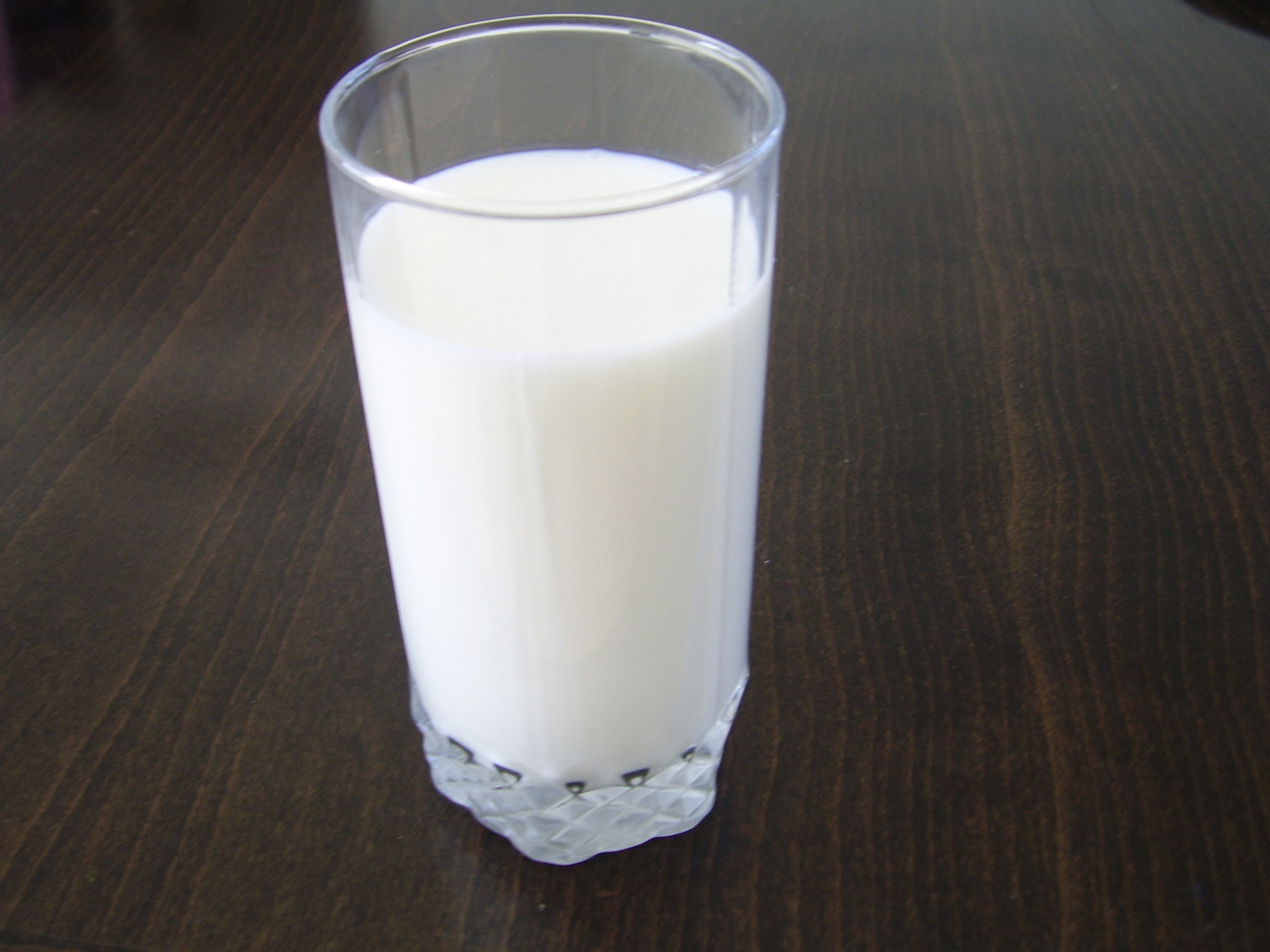One thing we cows know a lot about is milk. So, Fred let's start off by talking about how to measure milk. The main units for measuring liquids are:

• Millilitres (abbreviated mL)
• Litres (abbreviated L)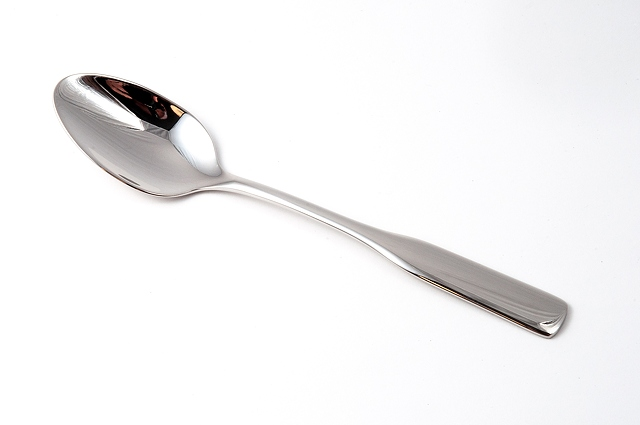The smallest of these units is the millilitre.

• You know that dropper that Farmer Jones uses to give you your eye drops? About $20$ drops of that will make up one millilitre.
• 5 millilitres of milk will fit into this teaspoon, so you can imagine how small a millilitre is.
• When I'm milked, I produce about $11$ mL of milk with each squirt.
• A glass of milk contains about $250$ mL of milk, and I'll produce about $350,000$ glasses of milk in my lifetime.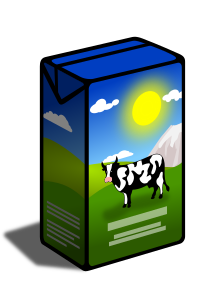Well millilitres definitely aren't enough to measure the amount of milk I produce each day. We need a bigger unit, and that is the litre (abbreviated as L). There are $1,000$ mL in $1$ L.

• My milk is sold in cartons at the supermarket. Each carton holds 1 litre.
• The milking bucket on the right holds about $10$ L of my milk. That's enough for $40$ glasses of milk!
• Fruit juice and other liquids are also sold in one or two litre cartons or bottles. Can you check them out for me next time you're at the supermarket and tell Fred what you've found out next time you come over to the farm?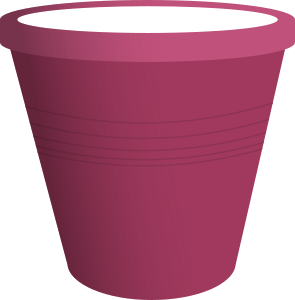## Measuring Mass (or Weight)

Many different foods like butter, cheese, yoghurt, and chocolate (yum!) are made using my milk. We measure the mass of these foods, and of course the mass of people and animals. We often call mass "weight", but weight is caused by gravity acting on mass, so it's a force.

There are three commonly used units of weight that you need to be familiar with, Fred:

• Grams
• Kilograms
• Tonnes

The smallest unit is the gram (abbreviated g), and the largest is the tonne (abbreviated t). Let's see what they're all about.Are you munching on raisins again, Fred? You'll spoil your dinner! How about eating an apple instead?

• Each of those raisins you're eating has a mass of about $1$ g.
• Small apples have a mass of around $150$ g.
• Butter is usually sold in $250$ g packets.
• No Fred, you're way too heavy for me to measure your mass in grams! We'll have to use bigger units.

The next biggest unit is the kilogram (abbreviated kg). There are

$1,000$ g in $1$ kg
Let's look at some other masses in kilograms.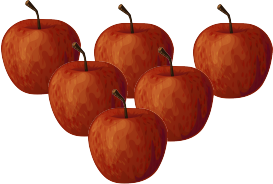• 6 small apples have a mass of 1 kg.
• Our friends here probably have masses around 40 kg.
• My mass is around 750 kg.

We measure mass using scales. There are different scales for measuring different ranges of masses. For example, kitchen scales measure masses in the range from 0 to 2,000 g. Bathroom scales measure masses in the range from 0 to 180 kg. Don't get on those kitchen scales, Fred! You'll break them!

Much heavier objects like cars, trucks and our friend Eric (the elephant) need to have their masses measured in tonnes. There are

$1,000$ kg in $1$ tonne.• Eric has a mass of about $5$ tonnes. t is short for tonne, so we can write this as $5$ t.
• A car has a mass of about $2$ t.

## Measuring Length

There are four common units for measuring length. From smallest to biggest, they are

• millimetres (abbreviated mm)
• centimetres (abbreviated cm)
• metres (abbreviated m)
• kilometres (abbreviated km)

### Millimetres• A pin (used in sewing) has a diameter of about $1$ mm.
• An Australian 10 cent piece is about $2$ mm thick.
• A New Zealand 10 cent piece is about $1.5$ mm thick.

### Centimetres

Carpenters make their measurements in millimetres, but most of the time, for slightly larger objects, we use centimetres. Remember that

$1$ cm is equal to $10$ millimetres.• A Christmas Beetle is about $2$ cm long.
• I am about $117$ cm tall.
• Our friend, Sam's desk is about $65$ cm wide.

### Metres

To measure the width of this field or a cricket oval, we need bigger units again. We use metres.

$1$ m is equal to $100$ cm, which is equal to $1,000$ mm.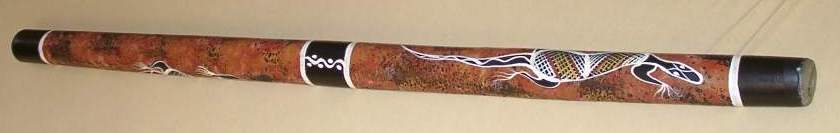• I'd like to learn to play the didgeridoo. This didgeridoo is about $1$ m long, but I'm looking for a slightly longer one as I'm told that longer didgeridoos make a deeper sound.
• My 4 year old friend, Timmy, is about $1$ metre tall.
• The Adelaide Oval is about $167$ m wide.

### KilometresIf you want to measure the distance between cities like Sydney and Perth, you need to use a bigger unit again. The preferred metric unit is the kilometre (abbreviated km).

$1$ km is equal to $1,000$ m.
Incidentally, the distance by car from Sydney to Perth is about $3,934$ km.

## Temperature

I took Fred to see the snow. He had fun playing in the snow, but he complained about how cold it was.We measure temperature using degrees Celsius (${}^\circ C$). Here are some common temperatures:

• Water freezes at $0^\circ C$. Snow is frozen water, so it needs to be below $0^\circ C$ for the snow to stick around, Fred. That's why it's so cold here at the snow.
• A hot summer's day might have a temperature of between $30^\circ C$ and $40^\circ C$.
• Water boils at $100^\circ$ Celsius.
• I set the oven to a temperature of $180^\circ C$ to make your favourite chocolate cake.

Well, that's it for our introduction to metric measurement. Thanks for your help! We'll talk more about these ideas in the article on metric units.

### Description

• Metric numbers
• Speed
• Conversions

and several other topics

### Audience

Year 10 or higher, some chapters are suitable for Year 8+ students

### Learning Objectives

Learn about measurement and related topics

Author: Subject Coach
You must be logged in as Student to ask a Question.